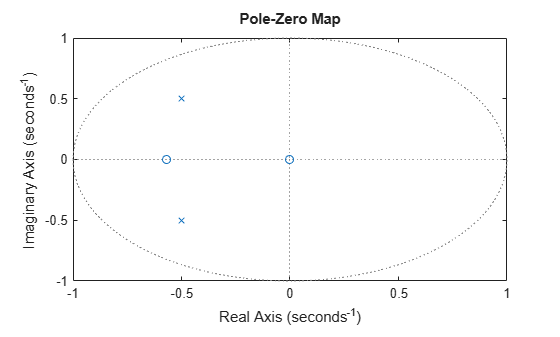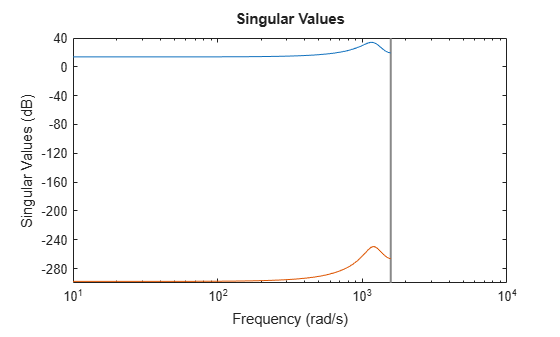# spectralfact

Spectral factorization of linear systems

## Syntax

``````[G,S] = spectralfact(H)``````
``````[G,S] = spectralfact(F,R)``````
``G = spectralfact(F,[])``

## Description

example

``````[G,S] = spectralfact(H)``` computes the spectral factorization:`H = G'*S*G`of an LTI model satisfying `H = H'`. In this factorization, `S` is a symmetric matrix and `G` is a square, stable, and minimum-phase system with unit (identity) feedthrough. `G'` is the conjugate of `G`, which has transfer function G(–s)T in continuous time, and G(1/z)T in discrete time.```

example

``````[G,S] = spectralfact(F,R)``` computes the spectral factorization:`F'*R*F = G'*S*G`without explicitly forming `H = F'*R*F`. As in the previous syntax, `S` is a symmetric matrix and `G` is a square, stable, and minimum-phase system with unit feedthrough.```

example

````G = spectralfact(F,[])` computes a stable, minimum-phase system `G` such that: `G'*G = F'*F`.```

## Examples

collapse all

Consider the following system.

```G0 = ss(zpk([-1 -5 1+2i 1-2i],[-100 1+2i 1-2i -10],1e3)); H = G0'*G0;```

`G0` has a mix of stable and unstable dynamics. `H` is a self-conjugate system whose dynamics consist of the poles and zeros of `G0` and their reflections across the imaginary axis. Use spectral factorization to separate the stable poles and zeros into `G` and the unstable poles and zeros into `G'`.

`[G,S] = spectralfact(H);`

Confirm that `G` is stable and minimum phase, by checking that all its poles and zeros fall in the left half-plane (`Re(s)` < 0).

`p = pole(G)`
```p = 4×1 complex 102 × -0.0100 + 0.0200i -0.0100 - 0.0200i -0.1000 + 0.0000i -1.0000 + 0.0000i ```
`z = zero(G)`
```z = 4×1 complex -1.0000 + 2.0000i -1.0000 - 2.0000i -5.0000 + 0.0000i -1.0000 + 0.0000i ```

`G` also has unit feedthrough.

`G.D`
```ans = 1 ```

Because `H` is SISO, `S` is a scalar. If `H` were MIMO, the dimensions of `S` would match the I/O dimensions of `H`.

`S`
```S = 1000000 ```

Confirm that `G` and `S` satisfy `H = G'*S*G` by comparing the original system to the difference between the original and factored systems. `sigmaplot` throws a warning because the difference is very small.

```Hf = G'*S*G; sigmaplot(H,H-Hf)```
```Warning: The frequency response has poor relative accuracy. This may be because the response is nearly zero or infinite at all frequencies, or because the state-space realization is ill conditioned. Use the "prescale" command to investigate further. ```Suppose that you have the following 2-output, 2-input state-space model, `F`.

```A = [-1.1 0.37; 0.37 -0.95]; B = [0.72 0.71; 0 -0.20]; C = [0.12 1.40 1.49 1.41]; D = [0.67 0.7172; -1.2 0]; F = ss(A,B,C,D);```

Suppose further that you have a symmetric 2-by-2 matrix, `R`.

```R = [0.65 0.61 0.61 -3.42];```

Compute the spectral factorization of the system given by `H = F'*R*F`, without explicitly computing `H`.

`[G,S] = spectralfact(F,R);`

`G` is a minimum-phase system with identity feedthrough.

`G.D`
```ans = 2×2 1 0 0 1 ```

Because `F` is has two inputs and two outputs, both `R` and `S` are 2-by-2 matrices.

Confirm that `G'*S*G = F'*R*F` by comparing the original factorization to the difference between the two factorizations. The singular values of the difference are far below those of the original system.

```Ff = F'*R*F; Gf = G'*S*G; sigmaplot(Ff,Ff-Gf)```Consider the following discrete-time system.

`F = zpk(-1.76,[-1+i -1-i],-4,0.002);`

F has poles and zeros outside the unit circle. Use `spectralfact` to compute a system `G` with stable poles and zeros, such that `G'*G = F'*F`.

`G = spectralfact(F,[])`
```G = -3.52 z (z+0.5682) ------------------ (z^2 + z + 0.5) Sample time: 0.002 seconds Discrete-time zero/pole/gain model. ```

Unlike `F`, `G` has no poles or zeroes outside the unit circle. `G` does have an additional zero at `z = 0`, which is a reflection of the unstable zero at `z = Inf` in `F`.

`pzplot(G)`Confirm that `G'*G = F'*F` by comparing the original factorization to the difference between the two factorizations. The singular values of the difference are far below those of the original factorization.

```Ff = F'*F; Gf = G'*G; sigmaplot(Ff,Ff-Gf)```## Input Arguments

collapse all

Self-conjugate LTI model, specified as a `tf`, `ss`, or `zpk` model. Self-conjugate means that is equal to its conjugate, `H` = `H'`. The conjugate `H'` is the transfer function H(–s)T in continuous time and H(1/z)T in discrete time.

`H` can be SISO or MIMO, provided it has as many outputs as inputs. `H` can be continuous or discrete with the following restrictions:

• In continuous time, `H` must be biproper with no poles or zeros at infinity or on the imaginary axis.

• In discrete time, `H` must have no poles or zeros on the unit circle.

`F` factor of the factored form ```H = F'*R*F```, specified as a `tf`, `ss`, or `zpk` model. `F` cannot have more inputs than outputs.

`R` factor of the factored form ```H = F'*R*F```, specified as a symmetric square matrix with as many rows as there are outputs in `F`.

## Output Arguments

collapse all

LTI factor, returned as a `tf`, `ss`, or `zpk` model. `G` is a stable, minimum-phase system that satisfies:

• `H = G'*S*G`, if you use the syntax ```[G,S] = spectralfact(H)```.

• `G'*S*G = F'*R*F`, if you use the syntax `[G,S] = spectralfact(F,R)`.

• `G'*G = F'*F`, if you use the syntax ```G = spectralfact(F,[])```.

Numeric factor, returned as a symmetric matrix that satisfies:

• `H = G'*S*G`, if you use the syntax ```[G,S] = spectralfact(H)```. The dimensions of `S` match the I/O dimensions of `H` and `G`.

• `G'*S*G = F'*R*F`, if you use the syntax `[G,S] = spectralfact(F,R)`. The size of `S` along each dimension matches the number of outputs of `F`.

## Tips

• `spectralfact` assumes that `H` is self-conjugate. In some cases when `H` is not self-conjugate, `spectralfact` returns `G` and `S` that do not satisfy `H = G'*S*G`. Therefore, verify that your input model is in fact self-conjugate before using `spectralfact`. One way to verify `H` is to compare `H` to ```H - H'``` on a singular value plot.

`sigmaplot(H,H-H')`

If `H` is self-conjugate, the ```H - H'``` line on the plot lies far below the `H` line.